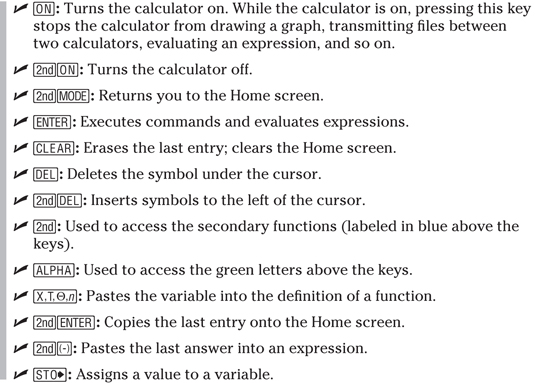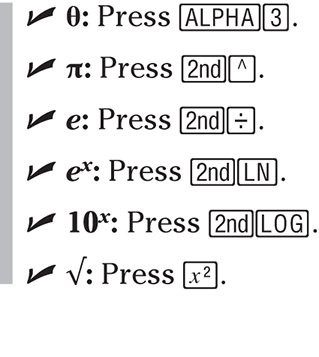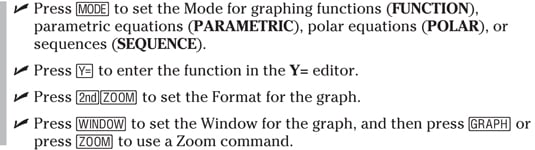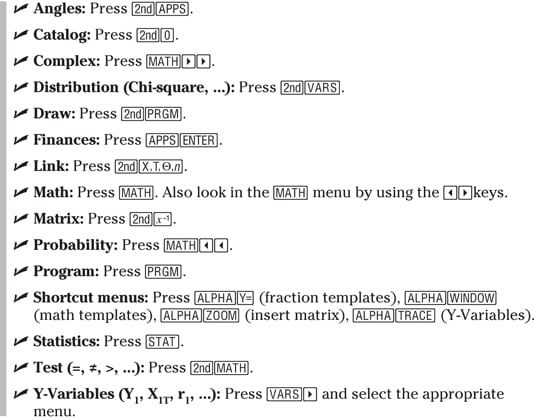##### TI-84 Plus CE Graphing Calculator For Dummies, 3rd EditionThe TI-84 Plus C graphing calculator is one of the most popular calculators in the world. Find out the important keystrokes you need to know to use the TI-84 Plus, and learn the math functions and constants that the TI-84 Plus C makes available to you.

Get to know the basics of graphing on your TI-84 Plus C, as well as how to use the calculator’s zoom commands and special menus.

## Important keystrokes

The TI-84 Plus C graphing calculator offers some very advanced functions, but to get to those advanced features, you have to know the basic keys to press. The keys to press most often appear in the following list:## Math functions and constants

Your TI-84 Plus C graphing calculator is quite capable of helping you out with math problems and actually comes equipped with ways to include constants and to input standard elements, such as π. The following list shows some of the more common and helpful math functions:## The basics of graphing on your TI-84 Plus C

You bought the TI-84 Plus C graphing calculator to help you do graphs, and help you it does. The points in the following list walk you through the steps to take to get your TI-84 Plus C to display a graph:## Special menus on the TI-84 Plus C

Not only does the TI-84 Plus C graphing calculator help you graph, but it also offers special menus you can use to look at distribution, probability, and even finances. The following list shows all the special menus you can access on the TI-84 Plus C:## Zoom commands

The TI-84 Plus C graphing calculator not only helps you make graphs, but its Zoom commands also help you highlight specific areas of a graph, redraw graphs in certain ratios, and otherwise tweak graphs to suit your needs. The following list shows you what each Zoom command does:

• ZBox: Draws a box around a portion of the graph and redraws the graph in a viewing window that has the dimensions of the box.

• Zoom In: Zooms in on the graph at the location of the cursor.

• Zoom Out: Zooms out on the graph at the location of the cursor.

• ZDecimal: Draws the graph in a –6.6 ≤ x ≤ 6.6 and –4.1 ≤ y ≤ 4.1 window. When the graph is traced, the x-coordinate of the Trace cursor equals an integral multiple of 0.1.

• ZSquare: Redraws the graph in a window that makes circles look like circles instead of ellipses.

• ZStandard: Draws the graph in a –10 ≤ x ≤ 10, –10 ≤ y ≤ 10 window.

• ZTrig: Draws the graph in a –11π/4 ≤ x ≤ 11π/4 and –4 ≤ y ≤ 4 window. When the graph is traced, the x-coordinate of the Trace cursor equals an integral multiple of π/24.

• ZInteger: Redraws the graph so that when it is traced, the x-coordinate of the Trace cursor equals an integer.

• ZoomStat: Finds an appropriate viewing window for stat plots.

• ZoomFit: Finds an appropriate viewing window for graphing functions, parametric equations, polar equations, or sequences.

• ZQuadrant1: This command graphs your function in a preset viewing window where 0 ≤ x ≤ 13.2 and 0 ≤ y ≤ 13.2. When you trace a function graphed in this window, the x-coordinate of the Trace cursor will be a multiple of 0.1.

• ZFrac1/2: This command graphs your function in a preset viewing window where –66/2 ≤ x ≤ 66/2 and –41/2 ≤ y ≤ 41/2. When you trace a function graphed in this window, the x-coordinate of the Trace cursor will be a multiple of 1/2.

• ZFrac1/3, ZFrac 1/4, ZFrac1/5, ZFrac 1/8, ZFrac1/10: These commands graph your function in a preset viewing window and work in the same manner as ZFrac1/2 does. If you think of d as the denominator of your fraction, then the viewing window is –66/dx ≤ 66/d and –41/dy ≤ 41/d. Tracing with these commands let you trace the x-coordinates by multiples of 1/d.